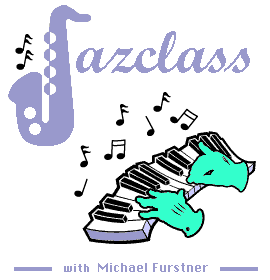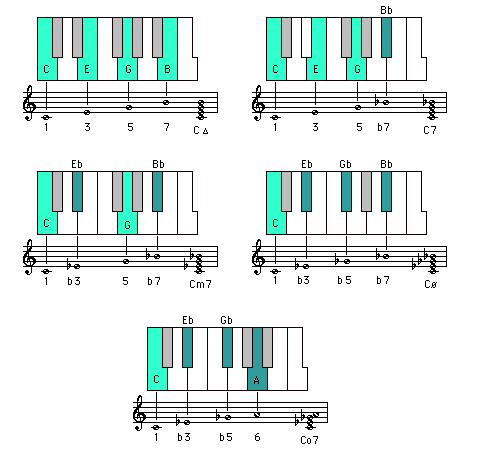Jazz Chords SEVENTH CHORD QUALITIES Description C | C# = Db | D | Eb | E | F | F# = Gb | G | Ab | A | Bb | B

Free Courses Online - Play-along Songs - My MP3s - My Blog - Jazclass Products - My Shop - Other

(Down - Top )

The five SEVENTH CHORD qualities

A seventh chord consists of 4 different notes.
When the root of the chord is at the bottom, all chord tones are stacked from the chord root upwards either on successive lines, or in successive spaces.

There are five qualities of seventh chords :

major 7th - dominant 7th - minor 7th - half diminished - diminished 7th

The major 7th chord is formed by the tonic (1st), 3rd, 5th and 7th note of the major scale wich starts on the chord root. For example the C major 7th chord is formed by the notes C, E, G and B.

 C1 D2 E3 F4 G5 A6 B7

The other chord qualities are derived from the major 7th chord.
To form from a major 7th chord (1 - 3 - 5 - 7) :

• a dominant 7th chord -> lower the 7th : 1 3 5 7 ---> 1 3 5 b7
(in C : C E G B ---> C E G Bb  = C7)

• a minor 7th chord -> lower the 3rd and 7th : 1 3 5 7 ---> 1 b3 5 b7
(in C : C E G B ---> C Eb G Bb  = Cm7)

• a half diminished chord -> lower the 3rd, 5th and 7th : 1 3 5 7 ---> 1 b3 b5 b7
(in C : C E G B ---> C Eb Gb Bb  = Cø)

• a diminished 7th chord -> lower the 3rd and 5th, and twice lower the7th : 1 3 5 7 ---> 1 b3 b5 6 (bb7)
(in C : C E G B ---> C Eb Gb A  = Co7)

Audio(Note : the Bbb of the Co7 chord is usually written as an 'A'.)

(
Down - Up - Top)

The five qualities of Seventh Chords in all 12 keys are listed below in Circle of Fifths order.

 C chords (Down - Up - Top) Cmaj7 = C - E - G - B C7    = C - E - G - Bb Cm7  = C - Eb - G - Bb Cø    = C - Eb - Gb - Bb Co7   = C - Eb - Gb - A G chords (Down - Up - Top) Gmaj7 = G - B - D - F# G7    = G - B - D - F Gm7  = G - Bb - D - F Gø    = G - Bb - Db - F Go7   = G - Bb - Db - E D chords (Down - Up - Top) Dmaj7 = D - F# - A - C# D7    = D - F# - A - C Dm7  = D - F - A - C Dø    = D - F - Ab - C Do7   = D - F - Ab - B A chords (Down - Up - Top) Amaj7 = A - C# - E - G# A7    = A - C# - E - G Am7  = A - C - E - G Aø    = A - C - Eb - G Ao7   = A - C - Eb - Gb E chords (Down - Up - Top) Emaj7 = E - G# - B - D# E7    = E - G# - B - D Em7  = E - G - B - D Eø    = E - G - Bb - D Eo7   = E - G - Bb - Db B chords (Down - Up - Top) Bmaj7 = B - D# - F# - A# B7    = B - D# - F# - A Bm7  = B - D - F# - A Bø    = B - D - F - A Bo7   = B - D - F - Ab F# chords (Down - Up - Top) F#maj7 = F# - A# - C# - E# (=F) F#7    = F# - A# - C# - E F#m7  = F# - A - C# - E F#ø    = F# - A - C - E F#o7   = F# - A - C - D# (F# is the enharmonic equivalent of Gb) Gb chords (Down - Up - Top) Gbmaj7 = Gb - Bb - Db - F Gb7    = Gb - Bb - Db - E Gbm7  = Gb - A - Db - E Gbø    = Gb - A - C - E Gbo7   = Gb - A - C - Eb C# chords (Down - Up - Top) C#maj7 = C# - E# (=F) - G# - B# (=C) C#7    = C# - E# (=F) - G# - B C#m7  = C# - E - G# - B C#ø    = C# - E - G - B C#o7   = C# - E - G - A# (C# is the enharmonic equivalent of Db) Db chords (Down - Up - Top) Dbmaj7 = Db - F - Ab - C Db7    = Db - F - Ab - B Dbm7  = Db - E - Ab - B Dbø    = Db - E - G - B Dbo7   = Db - E - G - Bb Ab chords (Down - Up - Top) Abmaj7 = Ab - C - Eb - G Ab7    = Ab - C - Eb - Gb Abm7  = Ab - B - Eb - Gb Abø    = Ab - B - D - Gb Abo7   = Ab - B - D - F Eb chords (Down - Up - Top) Ebmaj7 = Eb - G - Bb - D Eb7    = Eb - G - Bb - Db Ebm7  = Eb - Gb - Bb - Db Ebø    = Eb - Gb - A - Db Ebo7   = Eb - Gb - A - C Bb chords (Down - Up - Top) Bbmaj7 = Bb - D - F - A Bb7    = Bb - D - F - Ab Bbm7  = Bb - Db - F - Ab Bbø    = Bb - Db - E - Ab Bbo7   = Bb - Db - E - G F chords (Down - Up - Top) Fmaj7 = F - A - C - E F7    = F - A - C - Eb Fm7  = F - Ab - C - Eb Fø    = F - Ab - B - Eb Fo7   = F - Ab - B - D
(Up - Top - Jazclass Links)